The 5 Regular Polyhedra

 These 5 geometric figures are also known as the 5 Platonic Solids and are the only convex regular polyhedra that can exist. A regular polyhedron is defined as a solid three-dimensional object having faces where   • each face is a regular polygon. (A regular polygon has equal sides and equal angles).   • the same number of faces (or the same number of edges) meet at each vertex   • all the dihedral angles (the angles between the planes) are equal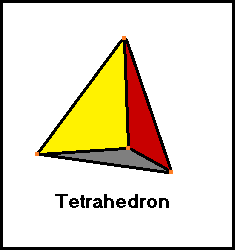3 faces and 3 edges meet at each vertex   Each face is an equilateral triangle.   4 faces   4 vertices   6 edges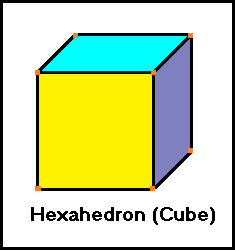3 faces and 3 edges meet at each vertex   Each face is a square.   6 faces   8 vertices   12 edges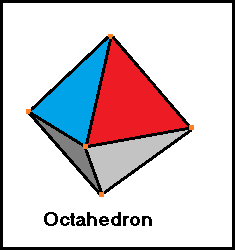4 faces and 4 edges meet at each vertex   Each face is an equilateral triangle.   8 faces   6 vertices   12 edges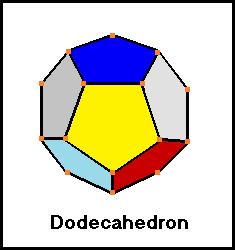3 faces and 3 edges meet at each vertex   Each face is a pentagon.   12 faces   20 vertices   30 edges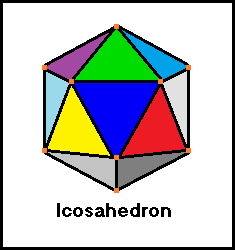5 faces and 5 edges meet at each vertex   Each face is an equilateral triangle.   20 faces   12 vertices   30 edges The Swiss mathematician Leonhard Euler (1707-1783) discovered the formula   V -E +F = 2   which states that the vertices minus the edges plus the faces of a convex polyhedron will always equal two. If we were to inscribe a sphere within any of the 5 Platonic solids, it would be tangent to the center of each face. If we were to circumscribe a sphere outside any of the 5 Platonic solids, it would pass through all of the vertices. C A L C U L A T O R >>>>> >>>>> >>>>> >>>>> >>>>> >>>>> >>>>> >>>>> >>>>> >>>>> >>>>> >>>>> P O L Y H E D R A     F O R M U L A S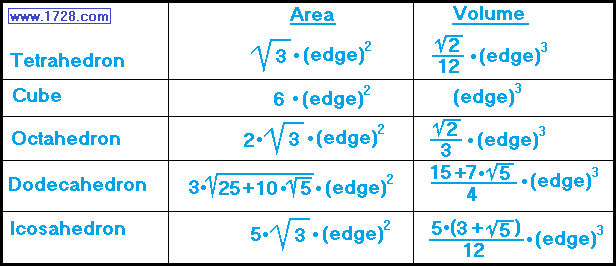Significant Figures >>> The default setting is for 5 significant figures but you can change that by inputting another number in the box above. Answers are displayed in scientific notation and for easier readability, numbers between .001 and 1,000 will be displayed in standard format (with the same number of significant figures.) The answers should display properly but there are a few browsers that will show no output whatsoever. If so, enter a zero in the box above. This eliminates all formatting but it is better than seeing no output at all. Return To Home Page Copyright © 1999 - 1728 Software Systems